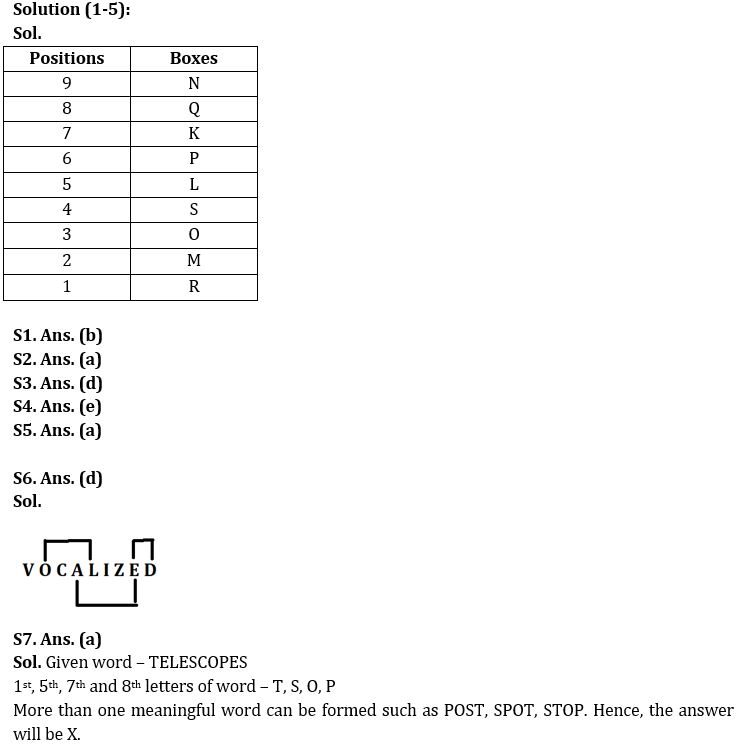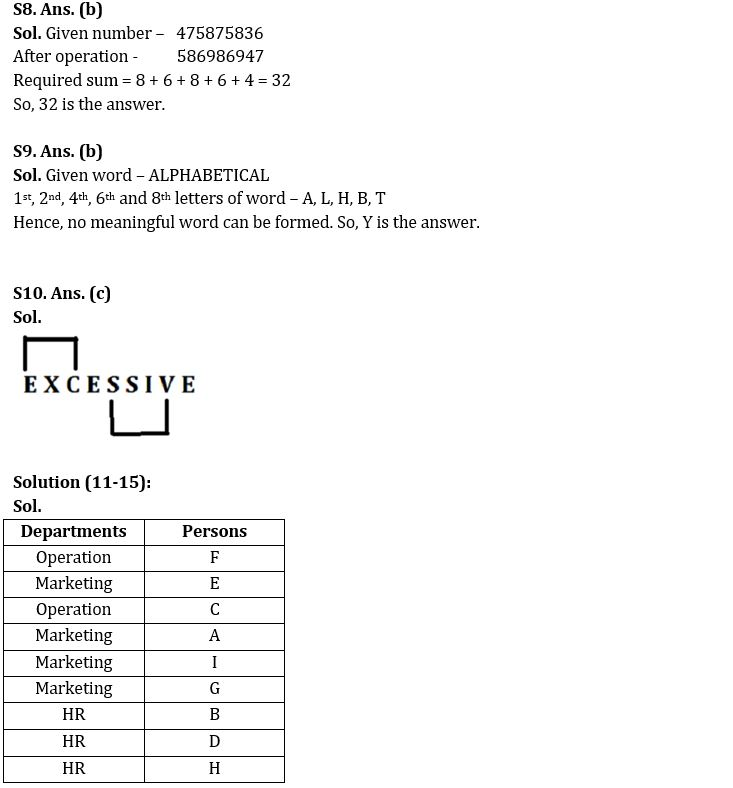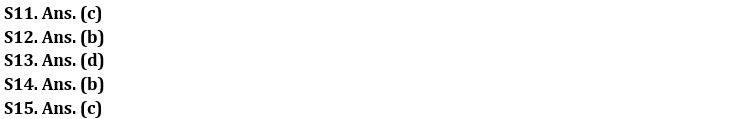Latest Banking jobs   »

# Reasoning Ability Quiz For IBPS RRB PO Clerk Prelims 2022- 17th August

Directions (1-5): Answer the questions based on the information given below.
Nine boxes K, L, M, N, O, P, Q, R and S are kept one above the other in such a way that bottommost box is numbered as 1, box above that is numbered as 2 and so on but not in the same order as given.
Two boxes are kept between P and N. S is kept three boxes above R which is an odd numbered box. O is the 3rd box from bottom. Number of boxes above K is one more than the number of boxes below M. M is kept below K. L is kept at prime number position. Q is kept two boxes above P.

Q1. Which box is kept at the bottommost position?
(a) M
(b) R
(c) N
(d) L
(e) None of these

Q2. How many boxes are between box R and M?
(a) None
(b) One
(c) Two
(d) Three
(e) None of these

Q3. Which box is kept three boxes above O?
(a) K
(b) L
(c) Q
(d) P
(e) None of these

Q4. Which box is kept at the topmost position?
(a) M
(b) R
(c) Q
(d) L
(e) None of these

Q5. Which box is kept exactly between K and O?
(a) L
(b) R
(c) Q
(d) P
(e) None of these

Q6. How many pairs of letters are there in the word ‘VOCALIZED’, which has as many letters between them as in English alphabetical series (both forward and backward direction)?
(a) One
(b) Four
(c) Two
(d) Three
(e) None of the above

Q7. If it is possible to make a meaningful word from 1st, 5th, 7th and 8th letters of word “TELESCOPES“, then which will be the 3rd letter of that meaningful word? If no such meaningful word can be formed, mark the answer as ‘Y’. If more than one meaningful word can be formed mark the answer as ‘X’.
(a) X
(b) Y
(c) S
(d) O
(e) T

Q8. If all the digits of the number ‘475875836’ are increased by 1, then what will be the sum of all the even digits of the new number after rearrangement?
(a) 36
(b) 32
(c) 28
(d) 40
(e) None of the above

Q9. If it is possible to make a meaningful word from 1st, 2nd, 4th, 6th and 8th letters of word “ALPHABETICAL“, then which will be the first letter of that meaningful word? If no such meaningful word can be formed, mark the answer as ‘Y’. If more than one meaningful word can be formed mark the answer as ‘X’.
(a) X
(b) Y
(c) H
(d) L
(e) B

Q10. How many pairs of letters are there in the word ‘EXCESSIVE’ which has as many letters between them as in English alphabetical series (both forward and backward direction)?
(a) One
(b) Four
(c) Two
(d) Three
(e) None of the above

Directions (11-15): Answer the questions based on the information given below.
9 persons (A, B, C, D, E, F, G, H and I) working in 3 different departments (Marketing, Operation and HR). At least two persons and at most four persons work in the same department.
Note: Person’s name with consecutive alphabets according to English alphabetical order doesn’t work in the same department.
E doesn’t work in operation. D and H works in same department. A doesn’t work with C who works with only 1 person. A and I work in same department but not in operation. F works in operation but not with H. G works in that department which has highest number of persons in it. B works with H but doesn’t work in marketing.

Q11. In which department does C work?
(a) HR
(b) Marketing
(c) Operation
(d) Either HR or operation
(e) Can’t be determined

Q12. In which department does A work and with how many persons?
(a) HR, three
(b) Marketing, three
(c) Marketing, two
(d) HR, two
(e) None of these

Q13. Who among the following works in same department as E?
(a) B
(b) C
(c) H
(d) I
(e) D

Q14. Who doesn’t work in HR department?
(a) D
(b) G
(c) H
(d) B
(e) Both D and H

Q15. Four from the following are similar in a certain way and forms a group. Find out the one which does not belong to that group.
(a) A, G
(b) C, F
(c) B, E
(d) D, H
(e) I, E

Solutions#### Congratulations!Download Hindu Review of October 2021: Free PDF